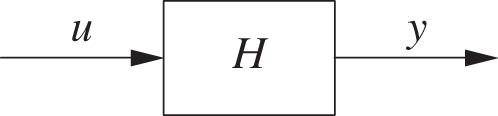## Chapter 21. Block-Diagram Algebra and the Feedback Equation

In Chapter 20 we saw that the dynamic behavior of a system is given as the solution to a differential equation. We also saw how the Laplace transform could be used to repackage all the dynamic information contained in a linear, time-invariant differential equation into a simple function (the transfer function). In this chapter, we will show how the dynamic behavior of a combination of systems can be found from the transfer functions of the individual elements.

## Composite Systems

In Chapter 20, we saw that, in the frequency domain, a system’s dynamic response y(s) to an external input u(s) is given by the product of the system’s transfer function H(s) and the input

y(s) = H(s)u(s)

We can express this equation as a block diagram, where the system (described by its transfer function H) transforms the input u to the output y:Obviously, we can combine several such systems in series, with the output of one serving as input to the next:The output of this composite system is the product of its components:

y(s) = H(s) G(s) u(s)

This follows simply because the output of the first element is x(s) = G(s) u(s) and because the output of the second component, acting on the output of the first, is y(s) = H(s) x(s). Therefore, the transfer function ...

Get Feedback Control for Computer Systems now with the O’Reilly learning platform.

O’Reilly members experience live online training, plus books, videos, and digital content from nearly 200 publishers.